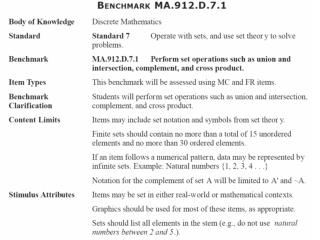# Algebra 1 Mini-Lessons - PowerPoint PPT PresentationDownload PresentationAlgebra 1 Mini-Lessons

Algebra 1 Mini-LessonsDownload Presentation## Algebra 1 Mini-Lessons

- - - - - - - - - - - - - - - - - - - - - - - - - - - E N D - - - - - - - - - - - - - - - - - - - - - - - - - - -
##### Presentation Transcript

1. Algebra 1 Mini-Lessons The set T represents several Taurine breeds of cattle. T {Angus, Devon, Shorthorn, Texas Longhorn} The set Z represents several Zebu breeds of cattle. Z {Boran, Nelore, Ponwar} What is the total number of elements in the set T X Z ? A. 7 B. 9 C. 12 D. 20 MA.912.D.7.1: Perform set operations such as union and intersection, complement, and cross product.

2. Algebra 1 Mini-Lessons If set A={-1,0,1} and set B={-3,-1,0,1,3}, what is the intersection of A and B? A. B. C. D. MA.912.D.7.1: Perform set operations such as union and intersection, complement, and cross product.

3. Algebra 1 Mini-Lessons Trina represents the girls in her class who play softball as set X and the girls in her class who play basketball as set Y Which notation should Trina use to represent all the girls who play both softball and basketball? A. B. C. D. MA.912.D.7.1: Perform set operations such as union and intersection, complement, and cross product.

4. Algebra 1 Mini-Lessons Three sets are defined below: Xis the set of all positive integers. Y is the set of all negative integers. Z is the set of all integers. Which of the following is true? A. B. C. D. MA.912.D.7.1: Perform set operations such as union and intersection, complement, and cross product.

5. Algebra 1 Mini-Lessons If A is the set ofall odd numbersand B is the set of perfect squares, which of these numbers is in the set of the union of A and B? 3 9 16 20 MA.912.D.7.1: Perform set operations such as union and intersection, complement, and cross product.

6. Algebra 1 Mini-Lessons If set , and , then which of the following could be set D? { blue} { blue, red, yellow} { red, yellow, orange, green} { blue, red, yellow, orange, green} MA.912.D.7.1: Perform set operations such as union and intersection, complement, and cross product.

7. Algebra 1 Mini-Lessons A community theater group is selling 100 raffle tickets for a fundraiser. The tickets are numbered 00–99, and only one ticket will be randomly drawn. Rafael wants to determine the probabilities of the events below. Event A: The winning ticket number will end in 0. Event B: The winning ticket number will begin with 5. Event C: The winning ticket number will end in 0 OR begin with 5. Event D: The winning ticket number will end in 0 AND begin with 5. Which of these describes the differences between Event C and Event D? Event D is a dependent event. Event C is an independent event. Event C is the union of Event A and Event B. Event D is the intersection of Event A and Event B. Event C is the intersection of Event A and Event B. Event D is the union of Event A and Event B. The probability of Event C is the difference between the probabilities of Event A and Event B. The probability of Event D is the sum of the probabilities of Event A and Event B. MA.912.D.7.1: Perform set operations such as union and intersection, complement, and cross product.

8. Algebra 1 Mini-Lessons Set D lists the ages of Dianna’s grandchildren. D = {2, 5, 6, 8, 10, 11} Set K lists the ages of Karen’s grandchildren. K = {2, 10, 18} Set P lists the ages of Patrick’s grandchildren. P = {10, 11, 14} What is the greatest age in the set (K P) D? Gridded Response: MA.912.D.7.1: Perform set operations such as union and intersection, complement, and cross product.